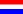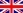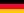You are not logged inFinitor
Independent measure and calculation system to make a map of a terrain. The data are retrieved from an encoded tachymetric or GPS measurement.
The format of the drawing file is dxf. Finitor has a range of functions to edit measurement data or coordinates.
€ 450,00
Order
Amount: Order
Tactio
Tactio is a program for automatic surveying with the Leica tachymeter TCRP1200 series of a plain cross section through a 3D-object. With cross section is meant the surface line, the profile, of the object. Automatic surveying means that a tachymeter (theodolite provided with a laser distance measuring device) on its own scans step by step the surface. The position of the tachymeter is independent of the choice of the plane.
Amount: Order
Transformation
The program searches for the best fit 3D transformation from one coordinate system to another. For example, a local tachymetric measured polygon can be transformed into an existing system. The corresponding coordinate points are in two separate text (csv) files.
The transformation is accomplished by the method of the least squares. The transformation concerns all coordinate points, not only the corresponding points. The transformed points are not deformed or rescaled.
€ 10,00
Order
Amount: Order
Regression
The program searches for the best fit straight line (or a circle, sphere, plane) through coordinates, using the method of least squares.
The coordinates are in a text (csv) file. De definition of the line and the distances are listed in a separate file.
€ 10,00
Order
Amount: Order
Neighbouring points
The program searches for xy coordinates which are within a specific distance of each other in a text file. The file records should have a csv format. The points found are marked and can be written to a separate file, to get an overview.
The program is meant to find doubles or wrong numbers.
€ 10,00
Order
Amount: Order
Intersection of two straight lines
The program calculates the intersection point of two straight lines. The straight lines are determined by chosen points from a text (csv) file.
If the straight lines are not in one plane, the intersection is determined as the middle of the shortest distance. The result is displayed directly and can be added to the existing coordinate points.
€ 0,00
Order
Amount: Order
TCO-COO
The program converts a tco file to a coo file and vice versa.
It is meant to automatically fit the record when exchanging between Finitor and another program.
€ 0,00
Order
Amount: Order
Renumber COO
The program renumbers the point numbers in a text (csv) file. It is meant to retrieve a new series of point numbers to avoid overwriting.
€ 0,00
Order
Amount: Order
Kolommen Verwisselen
This program alternates the x, y, z columns in a coordinates file. The file format is csv. The first column represents the point number. The second represents the x value. The third and fourth represent the y and the z value.
€ 0,00
Order
Amount: Order